Courses

# Physics Test 4 - Electrostatics, Capacitance, Current Electricity

## 25 Questions MCQ Test Mock Test Series for JEE Main & Advanced 2022 | Physics Test 4 - Electrostatics, Capacitance, Current Electricity

Description
This mock test of Physics Test 4 - Electrostatics, Capacitance, Current Electricity for JEE helps you for every JEE entrance exam. This contains 25 Multiple Choice Questions for JEE Physics Test 4 - Electrostatics, Capacitance, Current Electricity (mcq) to study with solutions a complete question bank. The solved questions answers in this Physics Test 4 - Electrostatics, Capacitance, Current Electricity quiz give you a good mix of easy questions and tough questions. JEE students definitely take this Physics Test 4 - Electrostatics, Capacitance, Current Electricity exercise for a better result in the exam. You can find other Physics Test 4 - Electrostatics, Capacitance, Current Electricity extra questions, long questions & short questions for JEE on EduRev as well by searching above.
QUESTION: 1

### Figure shows some of the electric field lines corresponding to an electric field. The figure suggests that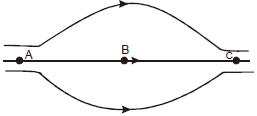Solution:

At point A and C, electric field lines are dense and equally spaced, so EA = EC
While at B they are far apart.
∴ EA = E> EB

QUESTION: 2

### When the separation between two charges is increased, the electric potential energy of the charges

Solution:

Potential  energy between two charges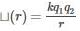Now if r increases and q1 and q2 are of same sign then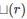decrases. if q1 and q2 are of oppositeincreases.
Therefore depending on signs of q1 and qmay increase or decrease.

QUESTION: 3

### An electric dipole is placed in a uniform electric field. The net electric force on the dipole

Solution: As dipole is combination of equal positive and negative charges, when an electric field is generated in the region of the dipole, torque can act on the dipole, but there will be no force on the dipole.
QUESTION: 4
Electric charge are distributed in a small volume. The flux of the electric field through a spherical surface of radius 10cm surrounding the total charge is 25V-m The flux over a concentric sphere of radius 20cm will be
Solution:
QUESTION: 5

A charge q is placed at the centre of the open end of a cylindrical vessel in figure. The flux of the electric field through the surface of the vessel is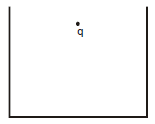Solution:

when a charge Q is placed at the centre of the open end of a cylindrical vessel then only half of the charge will contribute to the flux,because half will lie inside the surface and half will outside the surface so flux=Q/2ε0

QUESTION: 6
Mark the correct option
Solution:
QUESTION: 7

Two capacitors each having capacitance C and breakdown voltage V are joined in series. The capacitance and the breakdown voltage of the combination will be

Solution: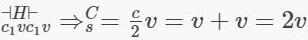QUESTION: 8

A dielectric slab is inserted between the plates of an isolated capacitor. The force between the plates will

Solution:
Here,the capacitor is isolated. It is already charged or else there would be no force. But when the dielectric is inserted, the charge remains fixed. If the capacitor were connected to a voltage source, then current would flow to keep the voltage fixed and then the force would increase.The voltage goes down even though there is no current in or out.The permittivity of any material is greater than the permittivity of free space. Therefore,When we insert the dielectric slab the capacitance rises.

QUESTION: 9

Two metal spheres of capacitances C1 & C2   carry some charges. They are put in contact and then separated. The final charges Q1  and Q2  on them will satisfy

Solution:
QUESTION: 10

Two resistances R and 2R are connected in parallel in an electric circuit. The thermal energy developed in R and 2 R are in the ratio

Solution:

Thermal energy = V2t/R
In parellel arrangement V is constant
So, for a time t
Thermal energy ∝ 1/R
Ratio of their thermal energy = R₂ : R₁ = 2R : R = 2 : 1

QUESTION: 11
A uniform wire of resistance 50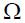is cut into 5 equal parts. These parts are now connected in parallel. The equivalent resistance of the combination is
Solution: Each part resistance is 10 ohm as R is directly proportional to length now 10 ohms 5 resistance are connected in parallel. Therefore 2 is equivalent resistance.
QUESTION: 12
The net resistance of an ammeter should be small to ensure that
Solution: Based on basic construction and implementation of ammeter,it is in connected in series in a circuit and should not appreciably change the current to be measured.
QUESTION: 13

The equivalent capacitance between the points A and B of five identical capacitors each of capacity C, will beSolution: The two identical branches capacitors have capacitance equal to C/2 each.
adding these in parallel will give you C/2 +C/2+C = 2C.
QUESTION: 14

The electric field intensity at a point situated 4 meters from a point charge is 200 N/C. If the distance is reduced to 2 meters, the field intensity will be

Solution:
QUESTION: 15

If an electron is brought towards an another electron, the electric potential energy of the system

Solution:
QUESTION: 16
Two identical conductors of a copper and aluminium are placed in an identical electric field. What is the magnitude of induced charge in the aluminium ?
Solution: As aluminum and copper are metals, their mobile electrons move under the influence of the external field until they reach the surface of the metal and collect there. External electric fields induce surface charges on metal objects that exactly cancel the field within. Since the field applied is same in both case, therefore induced charge will be the same. Since both are metals so equal amount of charge will induce on them.
QUESTION: 17

The electric field intensity at a point situated 4 meters from a point charge is 200 N/C. If the distance is reduced to 2 meters, the field intensity will be

Solution: 200=KQ/16-------equ-1
E=KQ/4-------------equ-2
on dividing1by 2
E= 800
QUESTION: 18

An electric dipole is placed at an angle of 30° with an electric field intensity 2 × 105 N/C. It experiences a torque equal to 4 N m. The charge on the dipole, if the dipole length is 2 cm, is

Solution:
QUESTION: 19

Shown in the figure is a distribution of charges. The flux of electric field due to these charges through the surface S is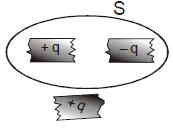Solution:

Flux flowing through a body depends on the net charge enclosed inside the surface, here net charge inside is zero so flux is also zero. And the third charge is outside so it will not effect the flux.

QUESTION: 20

Ten identical cells each of emf E and internal resistance r are connected in series to form a closed circuit. An ideal voltmeter connected across the three cells will read

Solution:

No. of cells = 10
Emf = E
Internal resistance = r
Let I = current through the ckt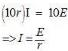Now potential (V) of across each cell will be
V = E -Ir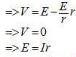We see there being no load resistance internal resistance play no effect on the observed emf
Thus the pd across 3 cells will be
E + E + E = 3Ir = 3E

*Answer can only contain numeric values
QUESTION: 21

A dipole consists of two particles one with charge +1µC and mass 1kg and the other with charge –1µC and mass 2kg separated by a distance of 3m. for small oscillations about its equilibrium position, the angular frequency, when placed in a uniform electric field of 20kV/m is :-

Solution:

Restoring torque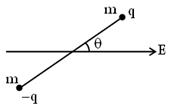τ = – PE sin θ
τ = – PE θ, for small θ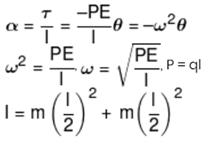*Answer can only contain numeric values
QUESTION: 22

A solid conducting sphere having a charge Q is surrounded by an uncharged concentric conducting hollow spherical shell. Let the potential difference between the surface of the solid sphere and that of the outer surface of the hollow shell be V. If the shell is now given a charge –3Q, the new potential difference between the same two surface is xV, then x is :-

Solution: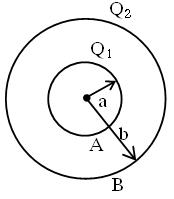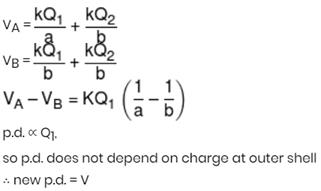*Answer can only contain numeric values
QUESTION: 23

A potentiometer is used for the comparison of e.m.f. of two cells E1 and E2. For cell E1 the no deflection point is obtained at 20 cm and for E2 the no deflection point is obtained at 30 cm. The ratio of their e.m.f.’s is N/3 then N is:

Solution:

Ratio will be equal to the ratio of no deflection lengths i.e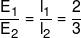*Answer can only contain numeric values
QUESTION: 24

The equivalent resistance of the arrangement of resistances shown in adjoining figure between the points A and B is N × 4 ohm then N is: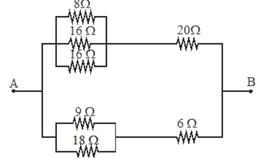Solution: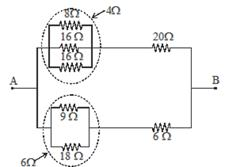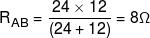*Answer can only contain numeric values
QUESTION: 25

In the circuit shown here C1 = 6μ F, C2 = 3μ F and battery B = 20V. The switch sis first closed. It is then opened and afterwards sis closed. What is the charge finally on C2.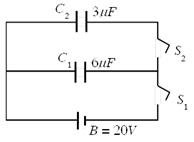Solution: